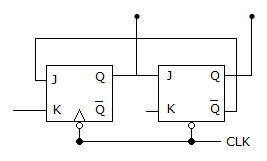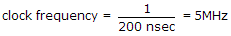# Electronics and Communication Engineering - Digital Electronics

21.

The modulus of counter in the given figure isA. 1 B. 2 C. 3 D. 4

Explanation:

Third clock pulse resets the counter to 00 state. Hence mod is 3.

22.

The input to a parity detector is 1001. The output is

 A. 0 B. 1 C. 0 or 1 D. indeterminate

Explanation:

Since the number of 1 's is even, output is 0.

23.

A 4 bit modulo 16 ripple counter uses JK flip-flops. If the propagation delay of each FF is 50 ns. The max. clock frequency that can be used is equal to

 A. 20 MHz B. 10 MHz C. 5 MHz D. 4 MHz

Explanation:

Propagation Delay for one FF is 50 nsec. For 4 FF = 50 x 4 = 200 nsec..

24.

As the number of flip flops are increased, the total propagation delay of

 A. ripple counter increases but that of synchronous counter remains the same B. both ripple and synchronous counters increase C. both ripple and synchronous counters remain the same D. ripple counter remains the same but that of synchronous counter increases

Explanation:

In ripple counter the clock pulses are applied to one flip- flop only.

Hence as the number of flip-flops increases the delay increases.

In synchronous counter clock pulses to all flip-flops are applied simultaneously.

25.

In a 4 input OR gate, the total number of High outputs for the 16 input states are

 A. 16 B. 15 C. 14 D. 13